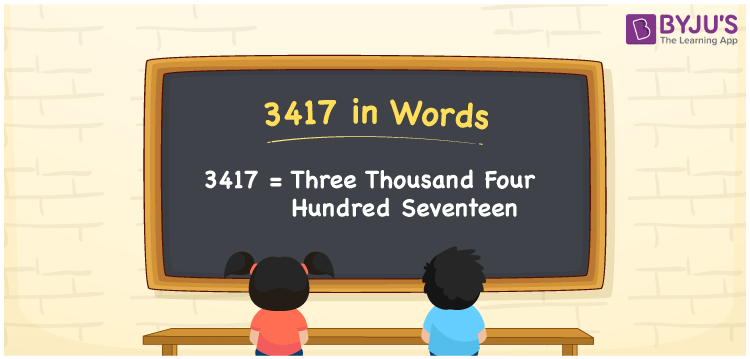3417 in words can be written as Three Thousand Four Hundred Seventeen. Students will be able to learn the conversion of 3417 in words which will help them understand the applications of numbers in our daily lives. If you buy a cooker for Rs. 3417, then you can say that “I have bought a cooker for Three Thousand Four Hundred Seventeen Rupees”. The  number 3417 can be written in words using the English alphabet. The numbers in words can be grasped easily by the students using the resources given at BYJU’S. 3417 in English can be read as “Three Thousand Four Hundred Seventeen”.

 3417 in words Three Thousand Four Hundred Seventeen Three Thousand Four Hundred Seventeen in Numbers 3417

## 3417 in English Words## How to Write 3417 in Words?

Students will learn about the conversion of 3417 into words from place value charts. The number 3417 has four digits. For 3417, the place value chart is prepared in a table form to help students understand it effectively.

 Thousands Hundreds Tens Ones 3 4 1 7

3417 in expanded form is explained in brief here:

3 × Thousand + 4 × Hundred + 1 × Ten + 7 × One

= 3 × 1000  + 4 × 100 + 1 × 10 + 7 × 1

= 3000 + 400 + 10 + 7

= 3417

= Three Thousand Four Hundred Seventeen

Therefore, 3417 in words is written as Three Thousand Four Hundred Seventeen.

3417 is a natural number that precedes 3418 and succeeds 3416.

3417 in words – Three Thousand Four Hundred Seventeen

Is 3417 an odd number? – Yes

Is 3417 an even number? – No

Is 3417 a perfect square number? – No

Is 3417 a perfect cube number? – No

Is 3417 a prime number? – No

Is 3417 a composite number? – Yes

## Frequently Asked Questions on 3417 in Words

Q1

### How do you write 3417 in words?

3417 can be written as “Three Thousand Four Hundred Seventeen” in words.
Q2

### Is 3417 an even number?

3417 is an odd number because it is not divisible by 2.
Q3

### How can Three Thousand Four Hundred Seventeen be written in numbers?

Three Thousand Four Hundred Seventeen can be written in numbers as 3417.